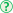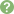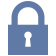Updated: 10/3/2020

# Statistical Distribution

0%
Topic
Review Topic
0
0
N/A
N/A
Questions
16
0
0
0%
0%
Evidence
5
0
0
Topic
 Descriptive Statistics Mean average of all observation mean = (sum of all observations)/(sample size) Median  the middle value of all observationsif sample size is odd median = ((n+1)/2)th largest value if the sample size is even median = the average of the (n/2)th and ((n/2)+1)th largest valueMode the most commonly occurring value if there is more than 1 most commonly occurring value, there are as many modes as most commonly occurring valuesin decreasing order of resistance to outliers, mode > median > meanTypes of Distributions Normal aka Gaussian, bell-shaped for continuous variables mean = median = mode Bi-modal distribution has 2 humps (each being a relative mode) if symmetrical, mean = median positive skew asymmetrical with tail trailing off to right mean > median > modenegative skew asymmetrical with tail trailing off to left mean < median < mode mean very sensitive to skewmedian somewhat resistant to skew mode very resistant to skew Other non-continuous variable types have their own distributions  e.g., binary, categorical, ordinal, binomial, and count variables
 Characteristics of the Normal Distribution For continuous variables Defined entirely by 2 parameters Mean (µ) standard deviation (σ) A certain percentage of all observations will always fall within +/- certain standard deviations of the mean+/- 1 standard deviation = 68%+/- 3 standard deviations = 99.7% Regression to the Mean Phenomenon in which sample points which were initially extreme often become closer to the mean in future measurements Most points will fall near on the average; therefore, extreme points are often a result of "luck" (e.g., a student performs particularly poor on an exam but normally performs at the average level) Has significance for study design e.g., patients with high blood pressure may improve after taking an experimental anti-hypertensive, but that improvement on the next measurement may be due to regression to the mean rather than the treatmentt the solution is to compare a control and experimental group. Measures of Variability Standard deviation a statistical measure that demonstrates how close together or spread apart the data is  if data is closer together, the standard deviation will be smaller (and vice versa) often designated by σ equation square root[(sum of the differences between each data point and the mean squared)/n] Standard error a statistical measure that demonstrates how far the sample mean is from the true population mean helps determine confidence intervals equation standard deviation/square root of n

Average 4.3 of 9 Ratings

Questions (16)Sorry, this question is forSorry, this question is forSorry, this question is forSorry, this question is forSorry, this question is for

(M1.ST.15.19) A study on cholesterol levels of a town in rural Idaho is performed, of which there are 1000 participants. It is determined that in this population, the mean LDL is 200 mg/dL, with a standard deviation of 50 mg/dL. If the population has a normal distribution, how many people have a cholesterol less than 300 mg/dL?

QID: 102598

680

6%

(4/62)

925

2%

(1/62)

950

21%

(13/62)

975

61%

(38/62)

997

3%

(2/62)

M 1 D

Select Answer to see Preferred Response

(M1.ST.14.17) On morning labs, a patient's potassium comes back at 5.9 mEq/L. The attending thinks that this result is spurious, and asks the team to repeat the electrolytes. Inadvertently, the medical student, intern, and resident all repeat the electrolytes that same morning. The following values are reported: 4.3 mEq/L, 4.2 mEq/L, and 4.2 mEq/L. What is the median potassium value for that patient that day including the first value?

QID: 103979

4.2 mEq/L

10%

(6/58)

4.25 mEq/L

76%

(44/58)

4.3 mEq/L

2%

(1/58)

4.65 mEq/L

3%

(2/58)

1.7 mEq/L

3%

(2/58)

M 1 E

Select Answer to see Preferred ResponseSorry, this question is for

(M1.ST.13.67) A clinical trial investigating a new biomedical device used to correct congenital talipes equinovarus (club foot) in infants has recently been published. The study was a preliminary investigation of a new device and as such the sample size is only 20 participants. The results indicate that the new biomedical device is less efficacious than the current standard of care of serial casting (p < 0.001), but the authors mention in the conclusion that it may be due to a single outlier--a patient whose foot remained uncorrected by the conclusion of the study. Which of the following descriptive statistics is the least sensitive to outliers?

QID: 103884

Mean

0%

(0/55)

Median

16%

(9/55)

Mode

62%

(34/55)

Standard deviation

13%

(7/55)

Variance

2%

(1/55)

M 1 E

Select Answer to see Preferred Response

(M1.ST.13.69) A case series is published on the duration of symptoms in children presenting to their primary medical doctor with suspected viral upper respiratory infection. Figure A is presented in the article. What is mean, median, and mode of the duration of symptoms in this case series?

QID: 103886
FIGURES:

Mean 8, median 7, mode 9

56%

(35/62)

Mean 7, median 5, mode 13

10%

(6/62)

Mean 8, median 8, mode 9

15%

(9/62)

Mean 7, median 8, mode 9

6%

(4/62)

Not enough information provided.

3%

(2/62)

M 1 E

Select Answer to see Preferred ResponseSorry, this question is for

(M1.ST.13.66) You are conducting a study on hypertension for which you have recruited 60 African-American adults. If the biostatistician for your study informs you that the sample population of your study is approximately normal, the mean systolic blood pressure is 140 mmHg, and the standard deviation is 7 mmHg, how many participants would you expect to have a systolic blood pressure between 126 and 154 mmHg?

QID: 103883

10 participants

5%

(11/219)

41 participants

16%

(35/219)

57 participants

63%

(139/219)

68 participants

4%

(9/219)

Not enough information provided.

7%

(16/219)

M 1 E

Select Answer to see Preferred ResponseSorry, this question is forSorry, this question is for